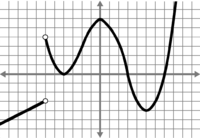# Analyzing graph related to f, f', and f''

#### peopsh8

##### New member1.
A) State the x−values over which g is increasing.

B) State the x−values over which g is decreasing.

C) State the x−values where g has horizontal tangent lines.

D) State the x−values over which g is differentiable.

2.
A) State the x−values over which g′ is positive.

B) State the x−values over which g′ is negative.

C) State the x−values over which g′ is zero.

D) State the x−values over which g′ is undefined.

Can someone please explain to me what the difference is.

#### tkhunny

##### Moderator
Staff member
The difference between what and what?

1.A and 2.A should produce identical responses.

It the same true of B, C, and D?

#### Jomo

##### Elite Member
g(x) I assume is the graph you posted. I can't be sure since there is no name of the graph given (it could be g(x), g'(x), g''(x) or some other graph)

Without you showing any work it is hard to answer your question but I'll try.

1) g(x) increasing: As you follow the graph going to the right, if g(x) is getting larger (going up) then the graph is increasing.

g(x) is decreasing: in the above definition just replace increasing with decreasing and larger with smaller.

g(x) has horizontal tangent line: You need to know what a tangent line is and what a horizontal line look likes.

2) g'(x)>0 is the set of all x-values where the slope of the tangent lines of g(x) are positive
g'(x)<0 is the set of all x-values where the slope of the tangent lines of g(x) are negative
g'(x)=0 is the set of all x-values where the slope of the tangent lines of g(x) is 0
g'(x) is undefined is the set of all x-values where the slope of the tangent lines do not exist.

3)g"(x) talks about the change in the slopes.
Always going left to right: If the slopes of the tangent lines are positive and getting less positive then the change in the slope is decreasing or negative. If the slopes of the tangent lines are negative and getting more negative then the change in the slope is decreasing or negative.
...

#### Jomo

##### Elite Member
You do realize your answering your questions as if you were given g(x). The problem is you were given g'(x).

There is a reason why we give different names to functions and that reason is so people can talk about the given function based on its name. Just because g'(x) is increasing over a certain interval does NOT mean that g(x) is increasing over that same interval.

Please redo the problem understanding that you are given the graph of g'(x) and post back.

#### peopsh8

##### New member
In my question I was asking the difference. I was just showing my solutions. Both of the functions a stated as g'. I don't understand why the solutions are what they are for number 1 when i read the graph from left to right.
As for number 2, my solutions are
a)(-oo,-6)u(-4,0)u(5,oo)
b)(-6,-4)u(0,5)
c)-4,0,5
d) -6
I collaborated with my tutor and they were still incorrect except d. I'm not understanding where we're going wrong.

#### Attachments

• 17.8 KB Views: 0

#### Jomo

##### Elite Member
The reason the solutions are what they are for number 1 when i read the graph from left to right is because you are reading the graph g(x)

If your tutor does not get why you are wrong then you need another tutor. There is no question about that.

Lets talk about C first. You said that I thought horizontal tangent lines were where the slope=0, (from what I gathered, usually at local maximums and minimums). This is true! Very good. So why is your answer wrong? You missed the point that the slope is 0. The slope is the derivative, g'(x). You want g'(x) = 0, NOT the slope of g'(x). g'(x) = 0 when x=-4 and x=3.

A) g is increasing when the slopes of g(x) are positive. But the slopes of g(x) are the values of g'(x). You want g'(x)>0. This happens when x is in (-6,3) U (7, infinity)

B) g is decreasing when the slopes of g are negative. That is when g'(x)<0. This happens when x is in (-infinity, -6) U (3,7).

D) You can compute g'(x) for any x-value except for x=-6 as g'(x) is not defined at x=-6 meaning the derivative is not defined for g(x).

Even if you did not know any calculus (because you were in say precalculus) I am confused why you would look at a function which is labelled g'(x) to see where it is increasing when you are asked to state where g(x) is increasing. These are two different functions which have two different graphs. When g(x) is decreasing there is no reason to think g'(x) is decreasing. They are different functions. When g(x) has a max point (slope is 0) there is no reason to think that g'(x) has a max point for the same x value. Why? Because they are different functions. Different functions can increase and decrease over different intervals. g(x) and g'(x) ar not the same function. The graph you were given was not named g(x). It was named g'(x). Am I getting through here?

#### peopsh8

##### New member
Thanks for the help

#### pka

##### Elite Member
View attachment 17569

So this is the solution for #1 and my attempt at answering.
My questions - I don't understand a) at all or b) -I thought horizontal tangent lines were where the slope=0, (from what I gathered, usually at local maximums and minimums)
To answer A) where is the graph of $$g^{\prime}>0$$
So $$g$$ is inreasing on $$(-6,-4)\cup (-4,3)\cup (7,\infty)$$. Note that points where $$g^{\prime}=0$$ are not included because if $$g^{\prime}=0$$ then $$g$$ is not increasing or decreasing.